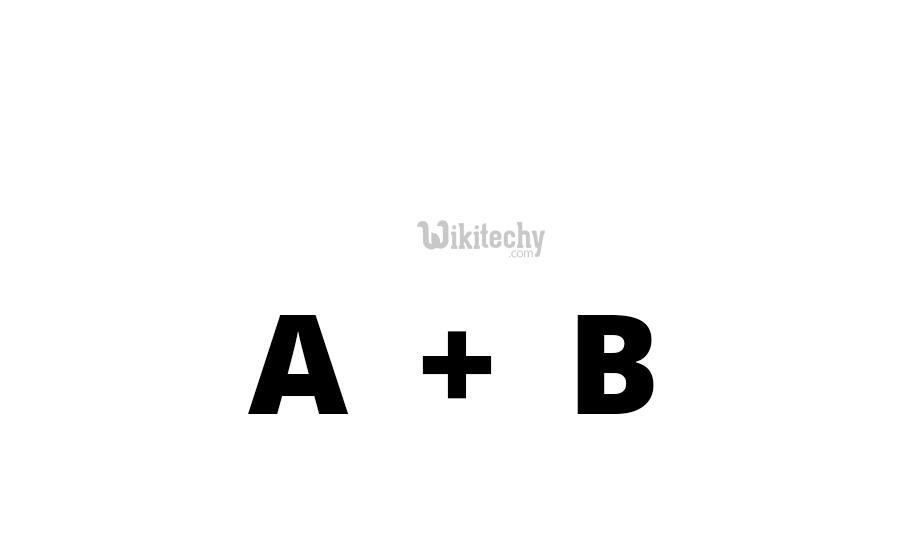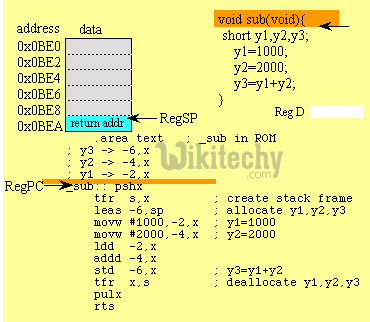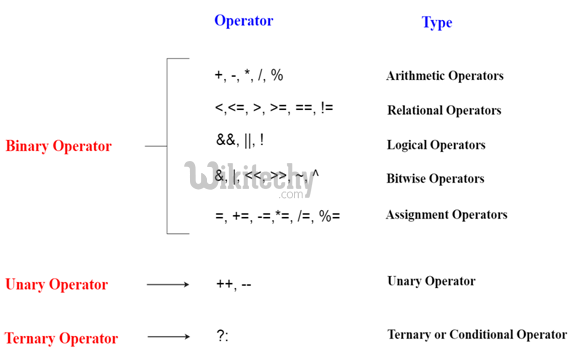# C# Operators - c# - c# tutorial - c# net

## What is an Operators in C# ?

• Expressions are constructed from operands and operators. The operators of an expression indicate which operations to apply to the operands. An operator is a special symbol which indicates a certain process is carried out. Operators in programming languages are taken from mathematics. The following table shows a set of operators used in the C# language.
• Arithmetic Operators
• Relational Operators
• Logical Operators
• Bitwise Operators
• Assignment Operators
• Conditional operators (ternary operators)
• Increment/decrement operators## Arithmetic Operators

• In C#-Programming the Arithmetic operators are used to perform mathematical calculationssuch as,
• Subtraction(-),
• Multiplication(*),
• Division(/) and
• Modulus(%).## Relational Operators:

• Relational operators are used to find the relation between two variables, i.e. to compare the values of two variables in a C# programming.## Logical Operators:

• The C# Logical Operator also evaluates the values and returns true or false as output.
• These based on true-false the program performs dynamically at a run time.
• This operator is generally used with C# programming.## Bitwise Operators:

• These operators are used to perform bit operations. Binary numbers are native to computers. Binary, octal, decimal, or hexadecimal symbols are only notations of the same number. Bitwise operators work with bits of a binary number. Bitwise operators are rarely used in higher level languages like C#.
• & (bitwise AND),
• | (bitwise OR),
• ~ (bitwise NOT),
• ^ (XOR),
• << (left shift) and </li>
• >> (right shift).

## Assignment Operators:

• In C# programs, values for the variables are assigned using assignment operators (=).

## Conditional operators (ternary operators)

• Conditional operators return one value if condition is true and returns another value is condition is false.
• Conditional operators is also known as ternary operator represented by the symbol " ? :" (question mark).

## Increment/decrement Operator:

• In C#- Programming Increment operators (++)is used to increase the value of the variable by one.
• In C#- Programming decrement operators (--)is used to decrease the value of the variable by one.